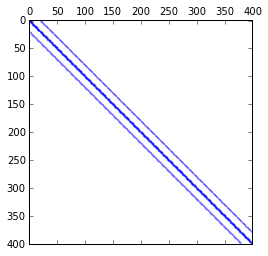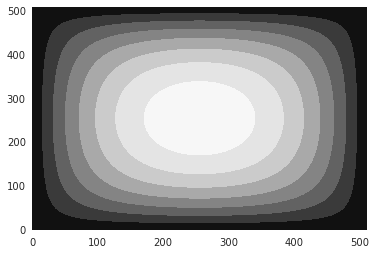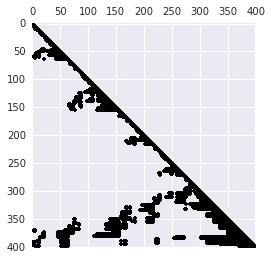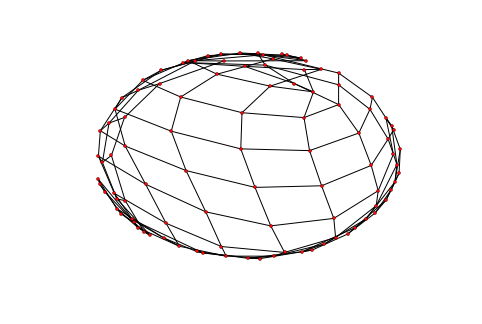# Lecture 11: From dense to sparse linear algebra¶

## Syllabus¶

Week 1: Matrices, vectors, matrix/vector norms, scalar products & unitary matrices
Week 2: TAs-week (Strassen, FFT, a bit of SVD)
Week 3: Matrix ranks, singular value decomposition, linear systems, eigenvalues
Week 4: Matrix decompositions: QR, LU, SVD + test + structured matrices start

## Recap of the previous lecture¶

• Algorithms for the symmetric eigenvalue problems (QR-algorithm, Divide-and-Conquer, bisection)
• SVD and its applications (collaborative filtering, integral equations, latent semantic indexing)

## Today lecture¶

Today we will talk about sparse matrices, where they arise, how we store them, how we operate with them.

• Formats: list of lists and compressed sparse row format, relation to graphs
• Fast matrix-by-vector product
• Fast direct solvers for Gaussian elimination

## Sparse matrices are ubiqitous in PDE¶

Consider the simplest partial differential equation (PDE), called Laplace equation:
$$\Delta T = \frac{\partial^2 T}{\partial x^2} + \frac{\partial^2 T}{\partial y^2} = f.$$

## Discretization¶

$$\frac{\partial^2 T}{\partial x^2} \approx \frac{T(x+h) + T(x-h) - 2T(x)}{h^2} + \mathcal{O}(h^4),$$

same for $\frac{\partial^2 T}{\partial y^2},$ and we get a linear system.
First, let us do one-dimensional case:

After the discretization of the one-dimensional Laplace equation with Dirichlet boundary conditions we have $$\frac{u_{i+1} + u_{i-1} - 2u_i}{h^2} = f_i,$$

or in the matrix form

$$A u = f,$$
               and ($n = 5$ illustration)

\begin{equation} A=-\frac{1}{h^2}\begin{bmatrix} 2& -1 & 0 & 0 & 0\\ -1 & 2 & -1 & 0 &0 \\ 0 & -1 & 2& -1 & 0 \\ 0 & 0 & -1 & 2 &-1\\ 0 & 0 & 0 & -1 & 2 \end{bmatrix} \end{equation}

The matrix is triadiagonal and sparse
(and also Toeplitz: all elements on the diagonal are the same)

## Block structure in 2D¶

In two dimensions, we get equation of the form
$$\frac{4u_{ij} -u_{(i-1)j} - u_{(i+1)j} - u_{i(j-1)}-u_{i(j+1)}}{h^2} = f_{ij},$$

or in the Kronecker product form

$$\Delta_2 = \Delta_1 \otimes I + I \otimes \Delta_1,$$

where $\Delta_1$ is a 1D Laplacian, and $\otimes$ is a Kronecker product of matrices.

For matrices $A$ and $B$ its Kronecker product is defined as a block matrix of the form $$[a_{ij} B]$$

In the block matrix form the 2D-Laplace matrix can be written in the following form:

$$A = -\frac{1}{h^2}\begin{bmatrix} \Delta_1 + 2I & -I & 0 & 0 & 0\\ -I & \Delta_1 + 2I & -I & 0 &0 \\ 0 & -I & \Delta_1 + 2I & -I & 0 \\ 0 & 0 & -I & \Delta_1 + 2I &-I\\ 0 & 0 & 0 & -I & \Delta_1 + 2I \end{bmatrix}$$

We can create this matrix using scipy.sparse package (actually this is not the best sparse matrix package)

In :
import numpy as np
import scipy as sp
import scipy.sparse
from scipy.sparse import csc_matrix
import matplotlib.pyplot as plt
%matplotlib inline
n = 20
ex = np.ones(n);
lp1 = sp.sparse.spdiags(np.vstack((ex,  -2*ex, ex)), [-1, 0, 1], n, n, 'csr');
e = sp.sparse.eye(n)
A = sp.sparse.kron(lp1, e) + sp.sparse.kron(e, lp1)
A = csc_matrix(A)
plt.spy(A, aspect='equal', marker='.', markersize=1)

Out:
<matplotlib.lines.Line2D at 0x10eccc390>## Sparsity pattern¶

The spy command plots the sparsity pattern of the matrix: the $(i, j)$ pixel is drawn, if the corresponding matrix element is non-zero.

Sparsity pattern is really important for the understanding the complexity of the sparse linear algebra algorithms.

Often, only the sparsity pattern is needed for the analysis of "how complex" the matrix is.

## Sparse matrix: formal definition¶

The formal definition of "sparse matrix" is that the number of non-zero elements is much less than the total number of

elements, so you can do the basic linear algebra operations (like solving linear systems at the first place) can be done faster,

than if working for with the full matrix.

The scipy.sparse package has tools for solving sparse linear systems:

In :
import numpy as np
import scipy as sp
import scipy.sparse
import scipy.sparse.linalg
import seaborn as sns
from scipy.sparse import csc_matrix, csr_matrix
import matplotlib.pyplot as plt
%matplotlib inline
n = 512
ex = np.ones(n);
lp1 = sp.sparse.spdiags(np.vstack((ex,  -2*ex, ex)), [-1, 0, 1], n, n, 'csr');
e = sp.sparse.eye(n)
A = sp.sparse.kron(lp1, e) + sp.sparse.kron(e, lp1)
A = csr_matrix(A)
rhs = np.ones(n * n)
sol = sp.sparse.linalg.spsolve(A, rhs)
plt.contourf(sol.reshape((n, n)))

Out:
<matplotlib.contour.QuadContourSet instance at 0x1020a6f38>## What we need to find out to see how it actually works¶

Question 1: How to store the sparse matrix in memory?

Question 2: How to solve linear systems with sparse matrices fast?

## Compressed sparse row (CSR)¶

A matrix is stored as 3 different arrays:

ia, ja, sa


where:

• ia is an integer array of length $n+1$
• ja is an integer array of length nnz
• sa is an real-value array of length nnz
• nnz is the total number of non-zeros for the matrix

## Idea behind CSR.¶

• For each row $i$ we store the column number of the non-zeros (and their) values
• We stack this all together into ja and sa arrays
• We save the location of the first non-zero element in each row

## CSR helps for matrix-by-vector product as well¶

for i in range(n):
for k in range(ia[i]:ia[i+1]):
y[i] += sa[k] * x[ja[k]]


Let us do a short timing test

In :
import numpy as np
import scipy as sp
import scipy.sparse
import scipy.sparse.linalg
from scipy.sparse import csc_matrix, csr_matrix, coo_matrix
import matplotlib.pyplot as plt
%matplotlib inline
n = 60
ex = np.ones(n);
lp1 = sp.sparse.spdiags(np.vstack((ex,  -2*ex, ex)), [-1, 0, 1], n, n, 'csr');
e = sp.sparse.eye(n)
A = sp.sparse.kron(lp1, e) + sp.sparse.kron(e, lp1)
A = csr_matrix(A)
rhs = np.ones(n * n)
B = coo_matrix(A)
%timeit A.dot(rhs)
%timeit B.dot(rhs)

10000 loops, best of 3: 46.7 µs per loop
10000 loops, best of 3: 56.8 µs per loop


As you see, CSR is faster, and for more unstructured patterns the gain will be larger.

## Sparse matrices and efficiency¶

Sparse matrix give complexity reduction.

But they are not very good for parallel implementation.

And they do not give maximal efficiency due to random data access.

Typically, peak efficiency of $10\%-15\%$ is considered good.

## How we measure efficiency of linear algebra operations¶

The standard way to measure the efficiency of linear algebra operations on a particular computing architecture is to

use flops (number of floating point operations per second)

The peak performance is determined as

frequency x number of cores x pipeline size x 2

We can measure peak efficiency of an ordinary matrix-by-vector product.

In :
import numpy as np
import time
n = 1000
a = np.random.randn(n, n)
v = np.random.randn(n)
t = time.time()
np.dot(a, v)
t = time.time() - t
print('Time: {0: 3.1e}, Efficiency: {1: 3.1e} Gflops'.\
format(t,  ((2 * n ** 2)/t) / 10 ** 9))

Time:  8.5e-04, Efficiency:  2.3e+00 Gflops

In :
n = 1000
ex = np.ones(n);
a = sp.sparse.spdiags(np.vstack((ex,  -2*ex, ex)), [-1, 0, 1], n, n, 'csr');
rhs = np.random.randn(n)
t = time.time()
a.dot(rhs)
t = time.time() - t
print('Time: {0: 3.1e}, Efficiency: {1: 3.1e} Gflops'.\
format(t,  (3 * n) / t / 10 ** 9))

Time:  1.4e-04, Efficiency:  2.2e-02 Gflops


## How to make things more efficient¶

Sparse matrix computations dominate the linear algebra computations nowdays.

They allow us to work with much larger matrices, but they utilize only $10\%-15\%$ percent of the peak computer performance.

It means, that our computer architecture is not well suited for standard sparse matrix algorithms.

There are many possible solutions of the problem, for example:

1. Use block sparse format
2. Reorder equations to make them more "block"
3. Instead of vectors, use "block vectors"
In :
n = 1000
k = 10
ex = np.ones(n);
a = sp.sparse.spdiags(np.vstack((ex,  -2*ex, ex)), [-1, 0, 1], n, n, 'csr');
rhs = np.random.randn(*(n, k))
t = time.time()
a.dot(rhs)
t = time.time() - t
print('Time: {0: 3.1e}, Efficiency: {1: 3.1e} Gflops'.\
format(t,  (3 * n * k) / t / 10 ** 9))

Time:  2.9e-04, Efficiency:  1.0e-01 Gflops


## Florida sparse matrix collection¶

There are many other types of matrices besides tridiagonal!

Florida sparse matrix collection which contains all sorts of matrices for different applications.

It also allows for finding test matrices as well!

We can have a look.

In :
from IPython.display import HTML
HTML('<iframe src=http://yifanhu.net/GALLERY/GRAPHS/search.html width=700 height=450></iframe>')

Out:

## Visualization of sparse matrices and graph¶

Sparse matrices and fast algorithms (especially for linear systems) have deep connection with graph theory.

First of all, sparse matrix can be treated as an adjacency matrix of a certain graph:

The vertices $(i, j)$ are connected, if the corresponding matrix element is non-zero.

Can you guess why it is important.

## Graph structure is important for LU decomposition¶

Why sparse linear systems can be solved fast?

Because the LU-decomposition is often also sparse (i.e., matrices $L$ and $U$ are sparse as well).

And how sparse they are, and which variables/equations select for the elimination at each step, is governed by the sparse structure.

And solving linear systems with sparse triangular matrices is very easy.

Note that the inverse matrix is not sparse!

In :
#Indeed, it is not sparse
n = 7
ex = np.ones(n);
a = sp.sparse.spdiags(np.vstack((ex,  -2*ex, ex)), [-1, 0, 1], n, n, 'csr');
a = a.todense()
b = np.array(np.linalg.inv(a))
print a
print b

[[-2.  1.  0.  0.  0.  0.  0.]
[ 1. -2.  1.  0.  0.  0.  0.]
[ 0.  1. -2.  1.  0.  0.  0.]
[ 0.  0.  1. -2.  1.  0.  0.]
[ 0.  0.  0.  1. -2.  1.  0.]
[ 0.  0.  0.  0.  1. -2.  1.]
[ 0.  0.  0.  0.  0.  1. -2.]]
[[-0.875 -0.75  -0.625 -0.5   -0.375 -0.25  -0.125]
[-0.75  -1.5   -1.25  -1.    -0.75  -0.5   -0.25 ]
[-0.625 -1.25  -1.875 -1.5   -1.125 -0.75  -0.375]
[-0.5   -1.    -1.5   -2.    -1.5   -1.    -0.5  ]
[-0.375 -0.75  -1.125 -1.5   -1.875 -1.25  -0.625]
[-0.25  -0.5   -0.75  -1.    -1.25  -1.5   -0.75 ]
[-0.125 -0.25  -0.375 -0.5   -0.625 -0.75  -0.875]]


## And the factors...¶

$L$ and $U$ are typically much better. In the tridiagonal case they are even bidiagonal!

In :
p, l, u = scipy.linalg.lu(a)
print l

[[ 1.          0.          0.          0.          0.          0.          0.        ]
[-0.5         1.          0.          0.          0.          0.          0.        ]
[-0.         -0.66666667  1.          0.          0.          0.          0.        ]
[-0.         -0.         -0.75        1.          0.          0.          0.        ]
[-0.         -0.         -0.         -0.8         1.          0.          0.        ]
[-0.         -0.         -0.         -0.         -0.83333333  1.          0.        ]
[-0.         -0.         -0.         -0.         -0.         -0.85714286
1.        ]]


## 2D-case¶

However, for a 2D case everything is much worser:

In :
n = 20
ex = np.ones(n);
lp1 = sp.sparse.spdiags(np.vstack((ex,  -2*ex, ex)), [-1, 0, 1], n, n, 'csr');
e = sp.sparse.eye(n)
A = sp.sparse.kron(lp1, e) + sp.sparse.kron(e, lp1)
A = csc_matrix(A)
T = scipy.sparse.linalg.spilu(A)
plt.spy(T.L, marker='.', color='k', markersize=8)

Out:
<matplotlib.lines.Line2D at 0x1159538d0>For two-dimensional case the number of nonzeros in the L factor grows as $\mathcal{O}(N^{3/2})$.

## Sparse matrices and graph ordering¶

The number of non-zeros in LU decomposition has a deep connection to the graph theory.

(I.e., there is an edge between $(i, j)$ if $a_{ij} \ne 0$.

networkx package can be used to visualize graphs, given only the adjacency matrix.

It may even recover to some extend the graph structure.

In :
import networkx as nx
n = 10
ex = np.ones(n);
lp1 = sp.sparse.spdiags(np.vstack((ex,  -2*ex, ex)), [-1, 0, 1], n, n, 'csr');
e = sp.sparse.eye(n)
A = sp.sparse.kron(lp1, e) + sp.sparse.kron(e, lp1)
A = csc_matrix(A)
G = nx.Graph(A)
nx.draw(G, pos=nx.spring_layout(G), node_size=10)## Fill-in minimization¶

Reordering the rows and the columns of the sparse matrix in order to reduce the number of non-zeros in $L$ and $U$ factors is called (fill-in minimization)

is based on graph theory:

• Minimum degree ordering - order by the degree of the vertex
• Cuthill–McKee algorithm (and reverse Cuthill-McKee) -- order for a small bandwidth
• Nested dissection: split the graph into two with minimal number of vertices on the separator

## Take home message¶

• CSR format for storage
• Sparse matrices & graphs ordering
• Ordering is important for LU fill-in

## Next week¶

• Iterative methods and preconditioners
• Matrix functions

# Questions?¶

In :
from IPython.core.display import HTML
def css_styling():